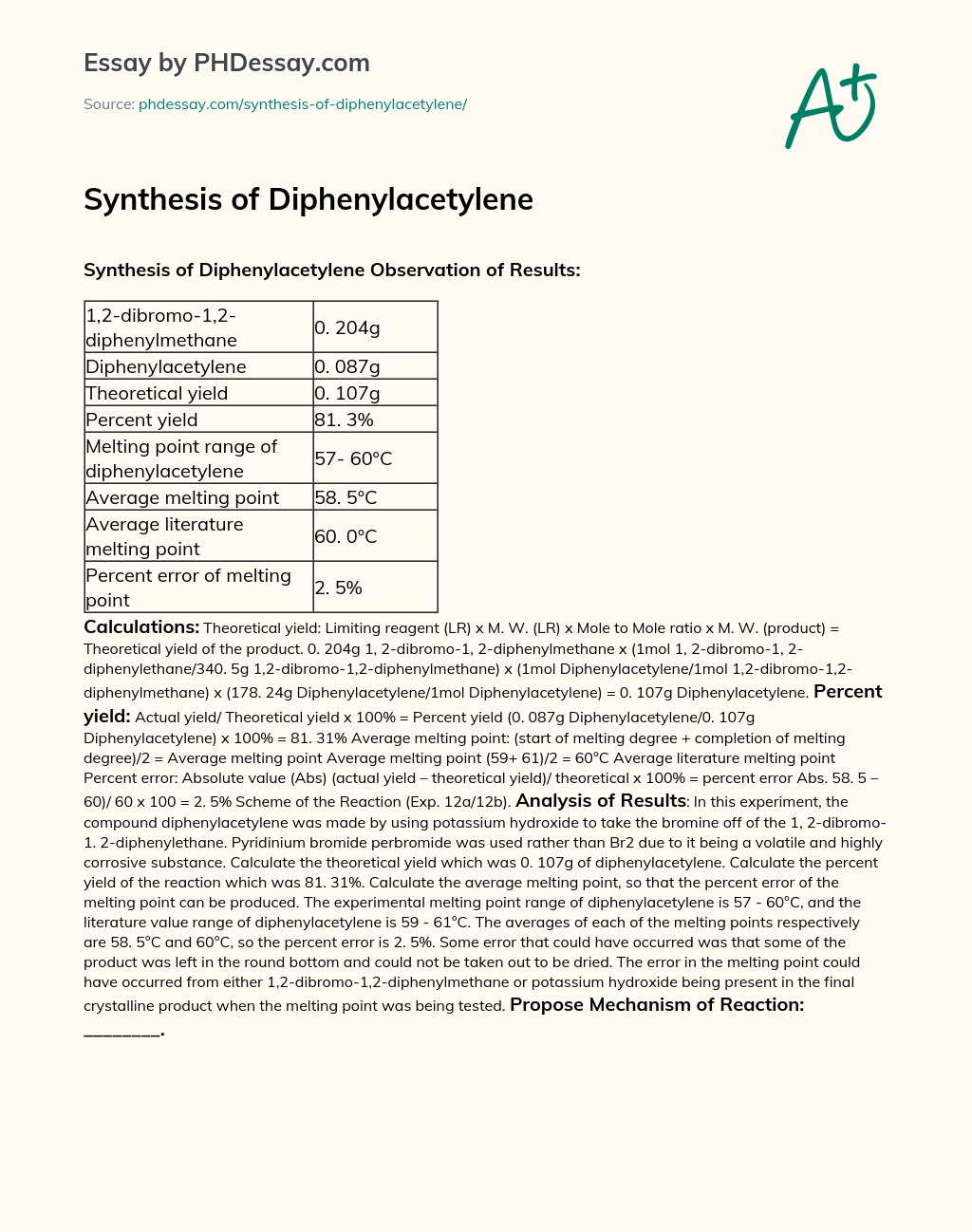Last Updated 20 Jul 2020

# Synthesis of Diphenylacetylene

Category Chemistry
Essay type Synthesis
Words 340 (1 page)
Views 170

### Synthesis of Diphenylacetylene Observation of Results:

Haven’t found the relevant content? Hire a subject expert to help you with Synthesis of Diphenylacetylene

Hire writer
 1,2-dibromo-1,2-diphenylmethane 0. 204g Diphenylacetylene 0. 087g Theoretical yield 0. 107g Percent yield 81. 3% Melting point range of diphenylacetylene 57- 60°C Average melting point 58. 5°C Average literature melting point 60. 0°C Percent error of melting point 2. 5%

Calculations: Theoretical yield: Limiting reagent (LR) x M. W. (LR) x Mole to Mole ratio x M. W. (product) = Theoretical yield of the product. 0. 204g 1, 2-dibromo-1, 2-diphenylmethane x (1mol 1, 2-dibromo-1, 2-diphenylethane/340. 5g 1,2-dibromo-1,2-diphenylmethane) x (1mol Diphenylacetylene/1mol 1,2-dibromo-1,2-diphenylmethane) x (178. 24g Diphenylacetylene/1mol Diphenylacetylene) = 0. 107g Diphenylacetylene.

Percent yield: Actual yield/ Theoretical yield x 100% = Percent yield (0. 087g Diphenylacetylene/0. 107g Diphenylacetylene) x 100% = 81. 31% Average melting point: (start of melting degree + completion of melting degree)/2 = Average melting point Average melting point (59+ 61)/2 = 60°C Average literature melting point Percent error: Absolute value (Abs) (actual yield – theoretical yield)/ theoretical x 100% = percent error Abs. 58. 5 – 60)/ 60 x 100 = 2. 5% Scheme of the Reaction (Exp. 12a/12b).

Analysis of Results: In this experiment, the compound diphenylacetylene was made by using potassium hydroxide to take the bromine off of the 1, 2-dibromo-1. 2-diphenylethane. Pyridinium bromide perbromide was used rather than Br2 due to it being a volatile and highly corrosive substance. Calculate the theoretical yield which was 0. 107g of diphenylacetylene. Calculate the percent yield of the reaction which was 81. 31%. Calculate the average melting point, so that the percent error of the melting point can be produced.

The experimental melting point range of diphenylacetylene is 57 - 60°C, and the literature value range of diphenylacetylene is 59 - 61°C. The averages of each of the melting points respectively are 58. 5°C and 60°C, so the percent error is 2. 5%. Some error that could have occurred was that some of the product was left in the round bottom and could not be taken out to be dried. The error in the melting point could have occurred from either 1,2-dibromo-1,2-diphenylmethane or potassium hydroxide being present in the final crystalline product when the melting point was being tested.

Propose Mechanism of Reaction: ________.Haven’t found the relevant content? Hire a subject expert to help you with Synthesis of Diphenylacetylene

Hire writer